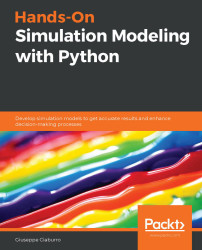•#### Hands-On Simulation Modeling with Python#### Overview of this book

Simulation modeling helps you to create digital prototypes of physical models to analyze how they work and predict their performance in the real world. With this comprehensive guide, you'll understand various computational statistical simulations using Python. Starting with the fundamentals of simulation modeling, you'll understand concepts such as randomness and explore data generating processes, resampling methods, and bootstrapping techniques. You'll then cover key algorithms such as Monte Carlo simulations and Markov decision processes, which are used to develop numerical simulation models, and discover how they can be used to solve real-world problems. As you advance, you'll develop simulation models to help you get accurate results and enhance decision-making processes. Using optimization techniques, you'll learn to modify the performance of a model to improve results and make optimal use of resources. The book will guide you in creating a digital prototype using practical use cases for financial engineering, prototyping project management to improve planning, and simulating physical phenomena using neural networks. By the end of this book, you'll have learned how to construct and deploy simulation models of your own to overcome real-world challenges.
PrefaceSection 1: Getting Started with Numerical SimulationFree Chapter
Chapter 1: Introducing Simulation ModelsChapter 2: Understanding Randomness and Random NumbersChapter 3: Probability and Data Generation ProcessesSection 2: Simulation Modeling Algorithms and TechniquesChapter 4: Exploring Monte Carlo SimulationsChapter 5: Simulation-Based Markov Decision ProcessesChapter 6: Resampling MethodsChapter 7: Using Simulation to Improve and Optimize SystemsSection 3: Real-World ApplicationsChapter 8: Using Simulation Models for Financial EngineeringChapter 9: Simulating Physical Phenomena Using Neural NetworksChapter 10: Modeling and Simulation for Project ManagementChapter 11: What's Next?Other Books You May Enjoy# Exploring deep neural networks

Deep learning is defined as a class of machine learning algorithms with certain characteristics. These models use multiple, hidden, nonlinear cascade layers to perform feature extraction and transformation jobs. Each level takes in the outputs from the previous level. These algorithms can be supervised, to deal with classification problems, or unsupervised, to deal with pattern analysis. The latter is based on multiple hierarchical layers of data characteristics and representations. In this way, the features of the higher layers are obtained from those of the lower layers, thus forming a hierarchy. Moreover, they learn multiple levels of representation corresponding to various levels of abstraction until they form a hierarchy of concepts.

The composition of each layer depends on the problem that needs to be solved. Deep learning techniques mainly adopt multiple hidden levels of an ANN but also sets of propositional formulas. The ANNs adopted have...